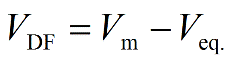PhysiologyWeb  Home  |  FAQ  |  About  |  Contact  |   - Sign InTweetElectrochemical Driving Force Calculator
When two or more ions contribute to the membrane potential across the plasma membrane (Vm) of a cell, it is likely that the membrane potential would not be at the equilibrium potential (Veq.) for any of the contributing ions. Thus, no ion would be at its equilibrium (i.e., Veq.Vm). When an ion is not at its equilibrium, an electrochemical driving force (VDF) acts on the ion, causing the net movement of the ion across the membrane down its electrochemical gradient. The driving force is quantified by the difference between the membrane potential and the ion equilibrium potential (VDF = VmVeq.). The magnitude of the driving force indicates how far an ion is from its electrochemical equilibrium. The arithmetic sign (i.e., positive or negative) of the driving force acting on an ion along with the knowledge of the valence of the ion (i.e., cation or anion) can be used to predict the direction of ion flow across the plasma membrane (i.e., into or out of the cell). See Table 1 below, and the lecture notes on the Resting Membrane Potential for additional details.
Electrochemical driving force equation• VDF is the electrochemical driving force acting on the ion of interest. Its value is generally reported in millivolts (mV). Its arithmetic sign (i.e., positive or negative) along with the knowledge of the valence of the ion (i.e., cation or anion) can be used to predict the direction of ion flow across the plasma membrane (i.e., into or out of the cell).
• Vm is the membrane potential. The membrane potential may be obtained by direct measurement, or may be predicted by using the Goldman-Hodgkin-Katz (GHK) equation.
• Veq. is the equilibrium potential for the ion of interest. Its value may be determined by using the Nernst equation.
Electrochemical driving force equation calculator
Each calculator cell shown below corresponds to a term in the formula presented above. Enter appropriate values in all cells except the one you wish to calculate. Therefore, at least two cells must have values, and no more than one cell may be blank. The value of the blank cell will be calculated based on the other values entered. After a calculation is performed, the calculated cell will be highlighted and subsequent calculations will calculate the value of the highlighted cell (with no requirement to have a blank cell). However, a blank cell has priority over a highlighted cell.
Please note that Vm, Veq., and VDF should all be expressed in the same unit (e.g., mV or V), however, they are usually expressed in millivolts (mV). The sign of the electrochemical driving force (VDF = VmVeq.) acting on any given ion allows us to determine the direction of ion flow – whether the ion moves into the cell, out of the cell, or exhibits no net movement across the plasma membrane. This information is provided after every calculation.
The membrane potential (Vm) may be obtained by direct measurement, or may be predicted by using the Goldman-Hodgkin-Katz (GHK) equation. The equilibrium potential (Veq.) for any ion may be determined by using the Nernst equation.
 Ionic species Cation Anion Equilibrium potential (Veq.) mV Membrane potential (Vm) mV Driving force (VDF = Vm − Veq.) mV
 Direction of ion flow:
Interpretation
Table 1. Sign of electrochemical driving force (VDF) and direction of ion flow
 Ionic species Sign of driving force(VDF = Vm − Veq.) Direction of ion flow Cation +0− OutwardNo net flowInward Anion +0− InwardNo net flowOutward
+ refers to VDF > 0, refers to VDF < 0, and 0 refers to VDF = 0. When VDF = 0, the ion is in electrochemical equilibrium. Cation, positively-charged ion; Anion, negatively-charged ion; Outward, ion movement out of the cell; Inward, ion movement into the cell.
Table 1 summarizes how the arithmetic sign of the driving force may be used to predict the direction of ion flow across the plasma membrane (i.e., into or out of the cell). For cations (positively charged ions such as Na+, K+, H+, and Ca2+), a positive driving force (i.e., VDF > 0) predicts ion movement out of the cell (efflux) down its electrochemical gradient, and a negative driving force (i.e., VDF < 0) predicts ion movement into the cell (influx). The situation is reversed for anions (negatively charged ions such as Cl and HCO3), where a positive driving force predicts ion movement into the cell (influx), and a negative driving force predicts ion movement out of the cell (efflux). If the membrane potential (Vm) is exactly at the equilibrium potential (Veq.) for an ion, the driving force acting on the ion would be zero. If Vm = Veq., it can be seen that VDF = VmVeq. = 0. In this case, the ion is said to be in electrochemical equilibrium, and there would be no net movement of the ion across the plasma membrane into or out of the cell (i.e., no net flux of ion).
See the lecture notes on the Resting Membrane Potential for additional details.

Posted: Monday, July 4, 2011
Last updated: Sunday, August 23, 2015